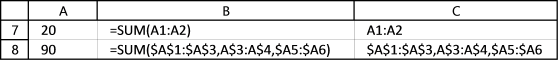Author: Oscar Cronquist Article last updated on February 02, 2018

I am trying to build a regular expression that matches cell references in a formula.

A regular expression is a sequence of characters that define a search pattern, according to Wikipedia.

This is the custom function I am using to extract cell references from a formula.

```
Function ExtractCellRefs(c As Range) As String

regexpattern = ""

With CreateObject("vbscript.regexp")
.Global = True
.MultiLine = True
.IgnoreCase = False
.Pattern = regexpattern
Set Results = .Execute(c.Formula)
End With

If Results.Count <> 0 Then
With Results
For d = 0 To .Count - 1
Rstr = Rstr & .Item(d) & ","
Next
End With
ExtractCellRefs = Left(Rstr, Len(Rstr) - 1)
Else
ExtractCellRefs = "No Matches"
End If

End Function

```

### Building the regular expression

The tricky part is the regular expression and I am a beginner at this, feel free to simplify my expression.

A cell reference can be anything from A1 to XFD1048576 so to match that the reg exp becomes:

[A-Z]{1,3}[0-9]{1,7}

[A-Z] matches any upper case letter from A to Z.

[A-Z]{1,3} matches 1 or up to 3 lower and upper case letters from A to Z. Example, XFD1048576 contains three letters.

[0-9]{1,7} matches 1 or more up to 7 digits from 0 to 9. Example, XFD1048576 contains 7 digits.

A cell reference can also be absolute or relative or both and the \$ sign tells which it is.

\\$?[A-Z]{1,3}\\$?[0-9]{1,7}

\ (backslash) escapes the character that follows

\\$ allows us to use the character \$, if I had not used the \ (backslash) \$ (dollar sign) had been taken for a match at end of string, I don't want that to happen.

\\$? the question mark matches zero or one of the pattern defined before it, in this case \$ (dollar sign)

The picture below shows the matches for above expression \\$?[A-Z]{1,3}\\$?[0-9]{1,7}It also works for this simple formula: =SUM(XFD1048576, \$A\$1,A\$1,\$A1), it returns these cell references: XFD1048576,\$A\$1,A\$1,\$A1

Cell reference to a cell range

The above regular expression finds only cell refs to a single cell, how do we find a solution to that?

A cell ref to a single cell looks like this =A1, a cell ref to a cell range may look like this =A1:C3

\\$?[A-Z]{1,3}\\$?[1-9]{1,7}(:\\$?[A-Z]{1,3}\\$?[1-9]{1,7})?

The new part is

(:\\$?[A-Z]{1,3}\\$?[0-9]{1,7})?

( (parentheses)  groups an expression

: a cell ref to a cell range contains a colon :

\\$?[A-Z]{1,3}\\$?[0-9]{1,7} is the same as before, it matches letters and digits

(:\\$?[A-Z]{1,3}\\$?[0-9]{1,7})  the parentheses groups the expression

(:\\$?[A-Z]{1,3}\\$?[0-9]{1,7})? the question mark matches zero or one of the pattern defined before it, in this case the groupCell references to other sheets

A cell reference to another sheet always ends with a ! (exclamation mark), the question mark matches zero or one of the pattern defined before it, in this case the ! (exclamation mark)

This is a new group so I am leaving out the previous expression for now, I will add it later. The regular expression is now !?

The sheet name may have lower and upper letters from A to Z and also numbers 0 to 9, the regular expression is [a-zA-Z0-9]{1,99}!?

If there is a blank space in the sheet name excel automatically surrounds the sheet name with two ' (apostrophe character), the expression becomes '?[a-zA-Z0-9]{1,99}'?!?

\s is any space character, this is what we have now '?[a-zA-Z0-9\s]{1,99}'?!?

'? the question mark matches zero or one of the pattern defined before it, in this case the ' (apostrophe character)Cell references to other workbooks

There may also be cell references to other workbooks, it would be nice to find them as well.

A reference to a cell range in another workbook has the workbook name surrounded by these characters [].

('?[a-zA-Z0-9\s\[\]]{1,99})?'?!? , \ (backslash) escapes the character that follows in this case \[\]

The file name has a dot between the file name and the extension, ('?[a-zA-Z0-9\s\[\]\.]{1,99})?'?!?The final expression is

('?[a-zA-Z0-9\s\[\]\.]{1,99})?'?!?\\$?[A-Z]{1,3}\\$?[0-9]{1,7}(:\\$?[A-Z]{1,3}\\$?[0-9]{1,7})?

### Final thoughts

I am sure there are characters allowed in a filename or sheet name that I have not considered in this post but I believe it will be easy to add those as well.

This does not take care of named ranges in a formula but it would not be hard to build a list of named ranges and then check if the formula contains named ranges.

Don't forget to use the regular expression in the UDF. Replace this line:

```regexpattern = ""
```

with this:

```regexpattern = "'?([a-zA-Z0-9\s\[\]\.])*'?!?\\$?[A-Z]+\\$?[0-9]+(:\\$?[A-Z]+\\$?[0-9]+)?"
```